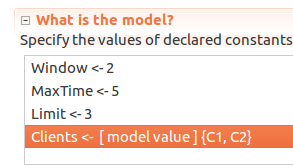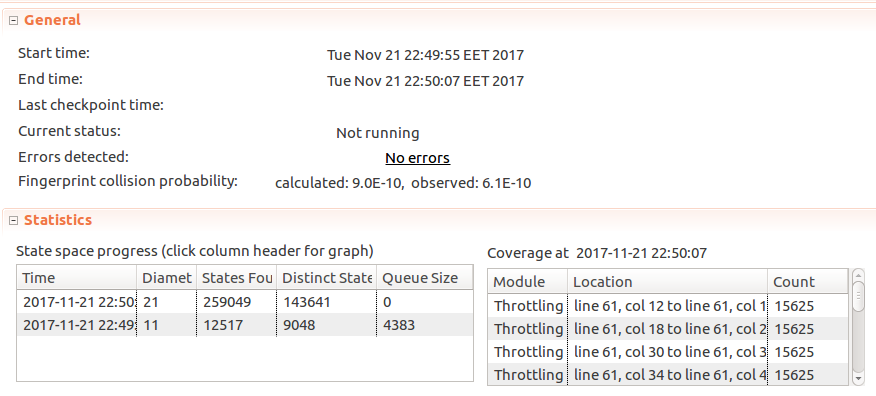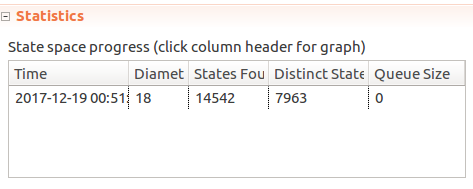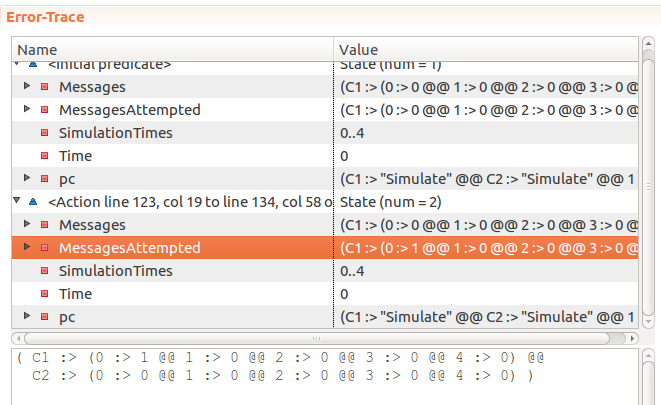Last time covered a basic specification of a single-sender message throttling algorith, which is nice, but in a real-world scenario you will usually have multiple clients interacting with the system, which is the idea for this post. The goal is to adapt our previous message throttling spec for multiple senders and explore it.

### The idea

In the previous spec the spec modelled having a single “message transmitter” and “message sender” as one entity, specified by a single program with a loop in it:

(* --algorithm throttling
\* ...
begin
Simulate:
while Time < MaxTime do
either
SendMessage:
ThrottledSendMessage(MessageId);
or
TimeStep:
Time := Time + 1;
end either;
end while;
end algorithm; *)


However, if we introduce multiple senders, it may be meaningful to control the number of senders, so we could have 1 “message transmitter” and N “message senders”. PlusCal has the concept of processes, which may help model the system in this case: transmitter and senders could be modelled as individual processes. Doing it this way would also allow for exploration of PlusCal processes as well.

So the idea here is to make the “Server” process just responsible for global time keeping. I don’t think there is a point in modelling inter-process message passing, or perhaps it’s an idea for future extension. “Client” processes would always attempt to send messages without waiting, TLC should take care of modelling distribution of messages in time.

### Spec

Now that there are multiple clients and we throttle per-client, we will need to keep track of senders in SendMessage:

macro SendMessage(Sender, Message)
begin
MessageLog := MessageLog \union {[Time |-> Time, Message |-> Message, Sender |-> Sender]};
end macro;


As mentioned above, the “Server” process can be responsible for just tracking time:

process Server = 1
begin
Simulate:
while Time < MaxTime do
Time := Time + 1;
end while;
end process;


So Server is defined as a PlusCal process that keeps incrementing the global Time variable. At the same time the clients can be defined as a process that just keeps attempting to send messages:

process Client \in Clients
variables MessageId = 0;
begin
Simulate:
while Time < MaxTime do
if SentMessages(self, GetTimeWindow(Time)) < Limit then
SendMessage(self, MessageId);
MessageId := MessageId + 1;
end if;
end while;
end process;


process Client \in Clients creates a process for each value in the Clients set. So if Clients = {1, 2, 3}, that will create 3 processes for each of the values. And self references the value assigned to the process. E.g. self = 1, self = 2 or self = 3. Macro body of ThrottledSendMessage has been inlined, as MessageId has been turned into a process local variable.

And lastly, the invariant needs to change to ensure that all clients have sent no more than the limited amount of messages in any window:

FrequencyInvariant ==
\A C \in Clients: \A T \in 0..MaxTime: SentMessages(C, GetTimeWindow(T)) <= Limit


Full PlusCal code:

----------------------------- MODULE Throttling -----------------------------
EXTENDS Naturals, FiniteSets
CONSTANTS Window, Limit, MaxTime, Clients

(* --algorithm throttling
variables
Time = 0,
MessageLog = {};

define
GetTimeWindow(T) ==
{X \in Nat : X <= T /\ X >= (T - Window)}

SentMessages(Sender, TimeWindow) ==
Cardinality({Message \in MessageLog: Message.Time \in TimeWindow /\ Message.Sender = Sender})
end define;

macro SendMessage(Sender, Message)
begin
MessageLog := MessageLog \union {[Time |-> Time, Message |-> Message, Sender |-> Sender]};
end macro;

process Server = 1
begin
Simulate:
while Time < MaxTime do
Time := Time + 1;
end while;
end process;

process Client \in Clients
variables MessageId = 0;
begin
Simulate:
while Time < MaxTime do
if SentMessages(self, GetTimeWindow(Time)) < Limit then
SendMessage(self, MessageId);
MessageId := MessageId + 1;
end if;
end while;
end process;

end algorithm; *)

FrequencyInvariant ==
\A C \in Clients: \A T \in 0..MaxTime: SentMessages(C, GetTimeWindow(T)) <= Limit

=============================================================================


Let’s try with a small model first: 2 clients, window of 2 seconds, modelled for 5 total seconds, with a limit of 3 seconds.Here client process names are defined as a set of model values. Model values are special values in TLA+ in that a value is only equal to itself and nothing else (C1 = C1, but C1 != C2, C1 != 5, C1 != FALSE, etc.), so it’s useful in avoiding mixing up with primitive types. Symmetry set can also be selected, as permutations in values of this set do not change the meaning: whether we have processes C1 and C2, or C2 and C1 - it doesn’t matter. Marking a set as a symmetry set, however, does allow TLC to reduce the search space and check specs faster.

When run with these values TLC doesn’t report any issuesLooking good! But so far the spec only checks if the number of messages sent is at or below the limit. But zero messages sent is also under the limit! So if SendMessage altered to drop all messages:

macro SendMessage(Sender, Message)
begin
MessageLog := {};
end macro;


And the model is rerun - TLC will get stuck on incrementing message IDs, but it should still pass. Not great! This fits the idea mentioned in the Amazon Paper, in which they suggest TLA+ forces one to think about what needs to go right. So what this model is still missing is at least to ensure if message can be sent - it is actually sent, not partially or incorrectly throttled.

But before going down that direction, there is a change to consider that would simplify the model and help resolve the infinite loop problem, thus helping demonstrate the message dropping issue more clearly.

#### Simplification

Hillel Wayne, author of the learntla.com, has recently helpfully suggested tracking number of messages sent using functions as opposed to tracking messages themselves, and counting them afterwards. It can be done because we don’t really care about message contents. Thanks for the tip!

To do that, Messages can be redefined as such:

variables
Time = 0,
SimulationTimes = 0..MaxTime,
Messages = [C \in Clients |-> [T \in SimulationTimes |-> 0]];


Each client will have a function, which returns another function holding the number of messages a Client has sent at a particular Time. Then addressing a specific time of a particular client can be as simple as Messages[Client][Time].

The way time windows are calculated needs to change as well, as this time TLC was unable to use a finite integer set instead of Naturals set:

Attempted to enumerate { x \in S : p(x) } when S:
Nat
is not enumerable
While working on the initial state:
/\ Time = 0
/\ Messages = ( C1 :> (0 :> 0 @@ 1 :> 0 @@ 2 :> 0 @@ 3 :> 0 @@ 4 :> 0) @@
C2 :> (0 :> 0 @@ 1 :> 0 @@ 2 :> 0 @@ 3 :> 0 @@ 4 :> 0) )
/\ pc = (C1 :> "Simulate" @@ C2 :> "Simulate" @@ 1 :> "Simulate_")
/\ SimulationTimes = 0..4


Here’s a response by Leslie Lamport detailing the issue a bit more. Rewritten GetTimeWindow as such:

GetTimeWindow(T) ==
{X \in (T-Window)..T : X >= 0 /\ X <= T /\ X >= (T - Window)}


To count the number of messages, summing with reduce can be convenient. For that I borrowed SetSum operator from the snippet Hillel recommended, ending up with:

TotalSentMessages(Sender, TimeWindow) ==
SetSum({Messages[Sender][T] : T \in TimeWindow})


The rest of the changes are trivial, so here’s the code:

----------------------------- MODULE ThrottlingSimplified -----------------------------
EXTENDS Naturals, FiniteSets, Sequences
CONSTANTS Window, Limit, MaxTime, Clients

(* --algorithm throttling
variables
Time = 0,
SimulationTimes = 0..MaxTime,
Messages = [C \in Clients |-> [T \in SimulationTimes |-> 0]];

define
GetTimeWindow(T) ==
{X \in (T-Window)..T : X >= 0 /\ X <= T /\ X >= (T - Window)}

Pick(Set) == CHOOSE s \in Set : TRUE

RECURSIVE SetReduce(_, _, _)
SetReduce(Op(_, _), set, value) ==
IF set = {} THEN value
ELSE
LET s == Pick(set)
IN SetReduce(Op, set \ {s}, Op(s, value))

SetSum(set) ==
LET _op(a, b) == a + b
IN SetReduce(_op, set, 0)

TotalSentMessages(Sender, TimeWindow) ==
SetSum({Messages[Sender][T] : T \in TimeWindow})

end define;

macro SendMessage(Sender)
begin
Messages[Sender][Time] := Messages[Sender][Time] + 1;
end macro;

process Server = 1
begin
Simulate:
while Time < MaxTime do
Time := Time + 1;
end while;
end process;

process Client \in Clients
begin
Simulate:
while Time < MaxTime do
if TotalSentMessages(self, GetTimeWindow(Time)) < Limit then
SendMessage(self);
end if;
end while;
end process;

end algorithm; *)

FrequencyInvariant ==
\A C \in Clients: \A T \in SimulationTimes: TotalSentMessages(C, GetTimeWindow(T)) <= Limit


And if we run the model, it passes:But if + 1 is removed from the SendMessage macro, effectively quietly dropping all messages - the model still passes. Gah!

#### Ensuring messages do get sent

The idea to fix this issue is simple - track message sending attempts. The invariant would then check if enough messages have been sent.

To do so, a new variable MessagesAttempted is added:

MessagesAttempted = [C \in Clients |-> [T \in SimulationTimes |-> 0]];


And an operator to count the total number of attempts made during a window:

TotalAttemptedMessages(Sender, TimeWindow) ==
SetSum({MessagesAttempted[Sender][T] : T \in TimeWindow})


Macro to mark the sending attempt:

macro MarkSendingAttempt(Sender)
begin
MessagesAttempted[Sender][Time] := MessagesAttempted[Sender][Time] + 1;
end macro;


And updated the client process to mark sending attempts:

process Client \in Clients
begin
Simulate:
while Time < MaxTime do
if TotalSentMessages(self, GetTimeWindow(Time)) < Limit then
SendMessage(self);
MarkSendingAttempt(self);
end if;
end while;
end process;


As for the invariant, there are two relevant cases: a) When there were fewer sending attempts than limit permits, all of them should be successfully accepted; b) When number of attempts is larger than the limit, number of messages successfully accepted should be at whatever Limit is set. Which could be written as such:

PermittedMessagesAcceptedInvariant ==
\A C \in Clients:
\A T \in SimulationTimes:
\/ TotalAttemptedMessages(C, GetTimeWindow(T)) = TotalSentMessages(C, GetTimeWindow(T))
\/ TotalAttemptedMessages(C, GetTimeWindow(T)) = Limit


Here’s the full code:

----------------------------- MODULE ThrottlingSimplified -----------------------------
EXTENDS Naturals, FiniteSets, Sequences
CONSTANTS Window, Limit, MaxTime, Clients

(* --algorithm throttling
variables
Time = 0,
SimulationTimes = 0..MaxTime,
Messages = [C \in Clients |-> [T \in SimulationTimes |-> 0]],
MessagesAttempted = [C \in Clients |-> [T \in SimulationTimes |-> 0]];

define
GetTimeWindow(T) ==
{X \in (T-Window)..T : X >= 0 /\ X <= T /\ X >= (T - Window)}

Pick(Set) == CHOOSE s \in Set : TRUE

RECURSIVE SetReduce(_, _, _)
SetReduce(Op(_, _), set, value) ==
IF set = {} THEN value
ELSE
LET s == Pick(set)
IN SetReduce(Op, set \ {s}, Op(s, value))

SetSum(set) ==
LET _op(a, b) == a + b
IN SetReduce(_op, set, 0)

TotalSentMessages(Sender, TimeWindow) ==
SetSum({Messages[Sender][T] : T \in TimeWindow})

TotalAttemptedMessages(Sender, TimeWindow) ==
SetSum({MessagesAttempted[Sender][T] : T \in TimeWindow})

end define;

macro SendMessage(Sender)
begin
Messages[Sender][Time] := Messages[Sender][Time] + 1;
end macro;

macro MarkSendingAttempt(Sender)
begin
MessagesAttempted[Sender][Time] := MessagesAttempted[Sender][Time] + 1;
end macro;

process Server = 1
begin
Simulate:
while Time < MaxTime do
Time := Time + 1;
end while;
end process;

process Client \in Clients
begin
Simulate:
while Time < MaxTime do
if TotalSentMessages(self, GetTimeWindow(Time)) < Limit then
SendMessage(self);
MarkSendingAttempt(self);
end if;
end while;
end process;

end algorithm; *)

FrequencyInvariant ==
\A C \in Clients: \A T \in SimulationTimes: TotalSentMessages(C, GetTimeWindow(T)) <= Limit

PermittedMessagesAcceptedInvariant ==
\A C \in Clients:
\A T \in SimulationTimes:
\/ TotalAttemptedMessages(C, GetTimeWindow(T)) = TotalSentMessages(C, GetTimeWindow(T))
\/ TotalAttemptedMessages(C, GetTimeWindow(T)) = Limit


If messages are successfully accepted, the model passes. However, if SendMessage is purposefully broken again by commenting out the incrementation, the model fails:

Invariant PermittedMessagesAcceptedInvariant is violated.Which is great, as this is exactly what we wanted to achieve.